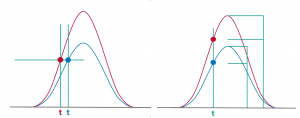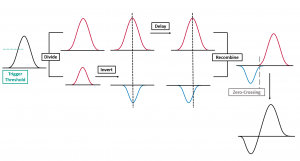Constant Fraction Discrimination | TCSPC

# Component Close-Up: Constant Fraction Discrimination

## Key Points

• Time-Correlated Single-Photon Counting (TCSPC) requires highly accurate and precise timing electronics.
• Excitation laser pulses and sample emission pulses can vary in height which can impact the correct timing.
• Constant fraction discrimination enables accurate timing despite pulse fluctuations and system noise.

## Leading Edge or Constant Fraction Discrimination

Accuracy in TCSPC is optimised by reducing the error in the recording of each individual photon event. One aspect of this is defining when the electronics record a time stamp. This is done using a discriminator which differentiates between “real” pulses and noise. Two common methods of discrimination are leading edge and constant fraction shown in Figure 1 where the difference in recorded pulse arrival time can be seen.Figure 1. Leading edge discrimination (left) where timing jitter is equal to the difference between the two readings of time, t. Constant fraction discrimination (right) where the time is taken at the same proportional height of the two incoming pulses.

The way in which constant fraction discrimination calculates amplitude independent pulse arrival time is demonstrated in Figure 2.Figure 2. Process of constant fraction discrimination where the incoming pulse is divided into 2/3 and 1/3, the lower portion inverted, the higher portion delayed, and the signals recombined. The zero-crossing point where the two portions of the signal combine is now independent of signal amplitude.1,2

Constant fraction discrimination is superior as it removes timing jitter from the data points by taking time stamps at the same relative height of the peak. This makes TCSPC insensitive to fluctuations in pulse amplitudes of the excitation light source, fluctuations of the detector output pulses, and to background noise of the detector. This is controlled by the threshold and the zero crossing levels.

For many detectors, the background (which also obeys Poisson statistics) is largely eliminated by the threshold allowing only pulses of a certain pulse amplitude (photon pulses) to be processed. The zero-crossing point renders all pulses passing the minimum voltage threshold independent of amplitude. This means that all sample photons will have consistent timings logged across the data set.

## References

1. R. Lakowicz, Principles of Fluorescence Spectroscopy Third Edition, 2010.
2. (Wolfgang) Becker, Advanced time-correlated single photon counting techniques, Springer, 2005.

Related ProductsFLS1000 Photoluminescence SpectrometerFS5 SpectrofluorometerMini-tau